# Models¶

The models are dictionaries with the properties of a machine or a calculation.

## Machine¶

Machines have a set of basic parameters, a stator, a magnet and a winding:

Parameter

Description

Unit

name

Name of machine

lfe

Lenght of iron

m

poles

Number of poles

outer_diam

Outer diameter (yoke side)

m

bore_diam

Bore diameter (airgap side)

m

inner_diam

Inner diameter (yoke)

m

airgap

airgap width

m

external_rotor

True, False

False

ffactor

processing factor for iron losses

dxffile

(see Model Creation with DXF)

### Stator¶

Stators have basic parameters and slots:

Parameter

Description

Default

num_slots

Number of Slots Q

num_slots_gen

Number of Slots in Model

m*Q/gcd(Q, 2p*m)

rlength

Relative iron length

1.0

mcvkey_yoke

Name of lamination material

dummy

mcvkey_teeth

Name of lamination material

dummy

nodedist

Factor for node distance

1.0

Note

if no value for num_slots_gen is given its value is calculated from the the number of slots Q and pole pairs p. (version added 0.0.16)

#### Stator Slots¶

Name

Parameter

stator1

slot_rf1, tip_rh1, tip_rh2, tooth_width, slot_width

stator2

slot_t1, slot_t2, slot_t3, slot_depth, slot_width, corner_width

statorRotor3

slot_height, slot_h1, slot_h2, slot_width, slot_r1, slot_r2, wedge_width1, wedge_width2, middle_line, tooth_width, slot_top_sh

stator4

slot_height, slot_h1, slot_h2, slot_h3, slot_h4, slot_width, slot_r1, wedge_width1, wedge_width2, wedge_width3

statorBG

yoke_diam_ins slot_h1, slot_h3, slot_width, slot_r1, slot_r2, middle_line, tooth_width, tip_rad, slottooth

<filename>

dxffile

Note

All units are metric units.

#### User defined Stator Slots with FSL¶

If a FSL file that includes the definition of stator geometry exists and is readable it can be used for the model creation.

Example with file mystator.fsl:

```machine = dict(
name="Motor",
...
stator=dict(
mcvkey_yoke='dummy',
mcvkey_shaft="dummy",
mystator=dict()
),
...
```

#### User defined Slots with DXF¶

If a DXF file that defines the stator geometry exists and is readable it can be used to create the FSL of the model. All DXF conversion parameters are supported.

Example:

```machine = dict(
name="Motor",
...
stator=dict(
mcvkey_yoke='dummy',
dxffile=dict(
name="mystator.dxf",
position='out',
split=True
)
),
...
```

Parameters

Description

Default

position

‘in’ or ‘out’

split

splits intersecting lines at their intersection-points

False

plot

creates the plot of the integrated object

False

– Note:: The split option is required only if intersecting lines have no common point.

### Windings¶

Name

Parameter

Default

num_phases

number of phases (m)

num_wires

number of wires per slot

coil_span

coil span

num_layers

number of layers

cufilfact

Fill factor of copper

0.45

culength

rel length of conductor

1.4

cuconduc

conductivity (S/m)

56e6

slot_indul

insulation thickness in slot

0.0

Windings may contain a leakage dict: leak_dist_wind, leak_evol_wind, leak_tooth_wind (version added 0.9.9)

• leak_dist_wind

Name

Parameter

Unit

m

m

endheight

End winding height

m

m.wiredia

Wire diameter

m

• leak_evol_wind

Name

Parameter

Unit

m

m

botlevel

Level at bottom of evolvents

m

toplevel

Level at top of evolvents

m

evolbend

m

endheight

End winding height

m

m.wiredia

Wire diameter

m

• leak_tooth_wind

Name

Parameter

Unit

m

endheight

End winding height

m

m.wiredia

Wire diameter

m

Example:

```windings=dict(
num_phases=3,
num_wires=100,
coil_span=3.0,
num_layers=1,
leak_dist_wind=dict(
endheight=20e-3,  # End winding height [m]
wiredia=1e-3)     # Wire diameter [m]
)
```

### Magnet¶

Magnets have basic parameters and slots:

Parameter

Description

Default

mcvkey_yoke

Name of lamination material

dummy

mcvkey_shaft

Name of shaft material

dummy

material

Name of magnet material

nodedist

Factor for node distance

1.0

Note

• the mcvkey parameters either reference a filename without extension (Example ‘M330-50A’) which must be found in the directory defined by the parameter magnetizingCurves of the Femag constructor or the name of an entry in the magnetizingCurve object.

• the material parameter references a name of the ‘Magnet Material’_ list.

#### Rotor Slots¶

Name

Parameter

magnetSector

magn_num, magn_width_pct, magn_height, magn_shape, bridge_height, magn_type, condshaft_r, magn_ori, magn_rfe, bridge_width, magn_len

magnetIron

magn_height, magn_width, gap_ma_iron, air_triangle, iron_height, magn_rem, condshaft_r, magn_ori, bridge_height, bridge_width, iron_shape

magnetIron2

magn_height, magn_width, gap_ma_iron, air_triangle, iron_height, magn_rem, condshaft_r, gap_ma_right, gap_ma_left, magn_ori, iron_shape

magnetIron3

magn_height, iron_bfe, gap_ma_iron, air_triangle, iron_height, gap_ma_right, gap_ma_left, condshaft_r, magn_num, magn_ori, iron_shape

magnetIron4

magn_height, magn_width, gap_ma_iron, iron_shape, air_space_h, iron_bfe, magn_di_ra, corner_r, air_sp_ori, magn_ori, magn_num

magnetIron5

magn_height, magn_width, gap_ma_iron, iron_bfe, air_space_h, corner_r, air_sp_ori, magn_num, iron_shape, air_space_b, magn_di_ra

magnetIronV

magn_height, magn_width, magn_angle, magn_num, iron_hs, iron_height, iron_shape, air_triangle, gap_ma_iron, magn_rem, condshaft_r

magnetFC2

yoke_height, iron_h1, iron_h2, iron_b, magn_width, magn_height, iron_bfe, iron_bfo, iron_shape, iron_hp, magn_num

<filename>

dxffile

Example:

```machine = dict(
name="PM 130 L4",
lfe=0.1,
poles=4,
outer_diam=0.13,
bore_diam=0.07,
inner_diam=0.015,
airgap=0.001,

stator=dict(
num_slots=12,
num_slots_gen=3,
mcvkey_yoke="dummy",
rlength=1.0,
stator1=dict(
slot_rf1=0.057,
tip_rh1=0.037,
tip_rh2=0.037,
tooth_width=0.009,
slot_width=0.003)
),

magnet=dict(
mcvkey_shaft="dummy",
mcvkey_yoke="dummy",
magnetSector=dict (
magn_num=1,
magn_width_pct=0.8,
magn_height=0.004,
magn_shape=0.0,
bridge_height=0.0,
magn_type=1,
condshaft_r=0.02,
magn_ori=2,
magn_rfe=0.0,
bridge_width=0.0,
magn_len=1.0 )
),

windings=dict(
num_phases=3,
num_wires=100,
coil_span=3.0,
num_layers=1)
)
```

#### User defined Magnet Slots with FSL¶

Example

If a FSL file that creates the magnet geometry exists and is readable it can be used for the model creation as an empty dict:

```machine = dict(
name="Motor",
...
magnet=dict(
mcvkey_yoke='dummy',
mcvkey_shaft="dummy",
myrotor=dict()
),
...
```

#### User defined Slots with DXF¶

If a DXF file that defines the magnet geometry exists and is readable it can be used to create the FSL for the model.

Example:

```machine = dict(
name="Motor",
...
magnet=dict(
mcvkey_yoke='dummy',
mcvkey_shaft="dummy",
dxffile=dict(
name='mymagnet.dxf',
position='in',
split=True
)
),
...
```

Parameters

Description

Default

position

‘in’ or ‘out’

split

splits intersecting lines at their intersection points

False

plot

creates the plot of the integrated object

False

– Note:: The split option is required only if intersecting lines have no common point.

## Magnetizing Curve¶

The MagnetizingCurve is a container of magnetizing curves (eg. lamination or PM material) that can be referenced by the model mcvkey attributes. It can either point to a directory of MC/MCV-File or hold a list of magnet curves which are identified by name.

Each magnetizing curve is described by the following properties

Attribute

Description

Unit

Default

name

Identifier of this curve

ctype

Type of curve

1

desc

Description

curve

List of dictionaries with bi (list of induction values) hi (List of field strength values) and angle which can be missing in case of 1 curve

T, A/m, deg

ch

hysteresis loss factor

0

cw

eddy current loss factor

0

ch_freq

hysteresis exponent

0

cw_freq

eddy-current exponent

0

b_coeff

induction loss exponent

0

Bo

reference induction

T

1.5

fo

reference frequency

Hz

50

fillfac

iron fill factor

1

bsat

saturation induction

T

2.15

rho

specific weight

kg/dm3

7.65

The loss factors and exponents are used in the Jordan loss calculation formula:

(cw*(f/fo)**cw_freq + ch*(f/fo)**ch_freq)*(B/Bo)**b_coeff

The Reader object which is included in the mcv module can be used to read MCV/MC files.

Permeability and polarisation calculation example:

```MUE0 = 4e-7*math.pi

r = mcv.get_results()

bh = [(bi, hi)
for bi, hi in zip(r['curve']['bi'],
r['curve']['hi']) if bi > 0 and hi > 0]

ji = [b-MUE0*h for b, h in bh]
muer = [bx/hx/MUE0 for bx, hx in bh]
```

Using a magnetizingcurve to write a mcv file:

```mcvData = dict(curve=[ dict(
bi=[0.0, 0.09, 0.179, 0.267, 0.358,
0.45, 0.543, 0.6334, 0.727,
0.819, 0.9142, 1.0142, 1.102,
1.196, 1.314, 1.3845, 1.433,
1.576, 1.677, 1.745, 1.787,
1.81, 1.825, 1.836],

hi=[0.0, 22.16, 31.07, 37.25, 43.174,
49.54, 56.96, 66.11, 78.291,
95, 120.64, 164.6, 259.36,
565.86, 1650.26, 3631.12, 5000, 10000,
15000, 20000, 25000, 30000, 35000, 40000]
)],
name='m270-35a',
desc=u"Demo Steel",
ch=4.0,
cw_freq=2.0,
cw=1.68)

mcv = femagtools.mcv.MagnetizingCurve(mcvData)

mcv.writefile('m270-35a')
```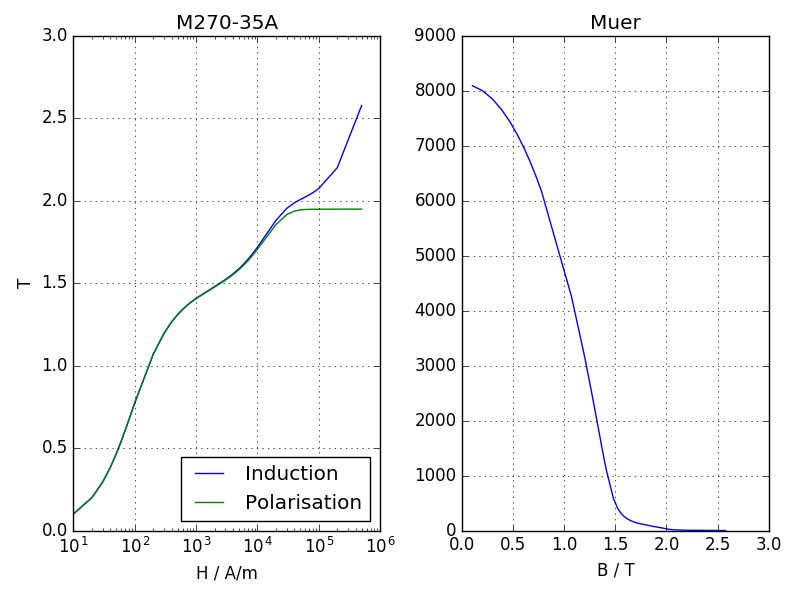Note

if the curve data is used in a stator or magnet slot model there is no need to create the file explicitly. Femagtools will take care of that during the model creation.

## Magnet Material¶

list of dict objects each having a unique name (or id) and a set of parameters that describe the magnet properties.

Parameter

Description

Default

Unit

name

Name of magnet material

mcvkey

name of nonlinear B(H) curve

orient

Magnetizing orientation

cartiso

rlen

Relative length

1.0

remanenc

Remanence Induction Br

T

relperm

Relative Permeability

spmaweight

Specific Mass

7500

kg/m³

temcoefbr

Temperature Coefficient of Br

-0.001

1/K

temcoefhc

Temperature Coefficient of Hc

-0.001

A/m/K

magntemp

Magnet Temperature

20

°C

magncond

Electr. Conductivity

625000

S/m

magnwidth

Magnet width

0.0

m

magnlength

Magnet length in z direction

0.0

m

Note

• name must be unique within list. It may be used as reference in the magnet model of the machine.

Example:

```magnets = [dict(name='MX-333', remanenc=1.2, relperm=1.05)]
```
• mcvkey is used for material that have a non-linear BH curve.

• the key orient describes the field orientation (mcartiso, mpoliso, martaniso, mpolaniso)

• rlen defines the relative length

Example:

```magnets=[dict(name='BH53M', mcvkey='BH53M',
orient='mcartiso', rlen=1.0)]
```
• The mcvkey can either reference a file or an entry in the magnetizing curve dict.

## User Specified Magnet Geometries¶

Magnet geometries not covered by the built in models can be specified by fsl code. Here is a example: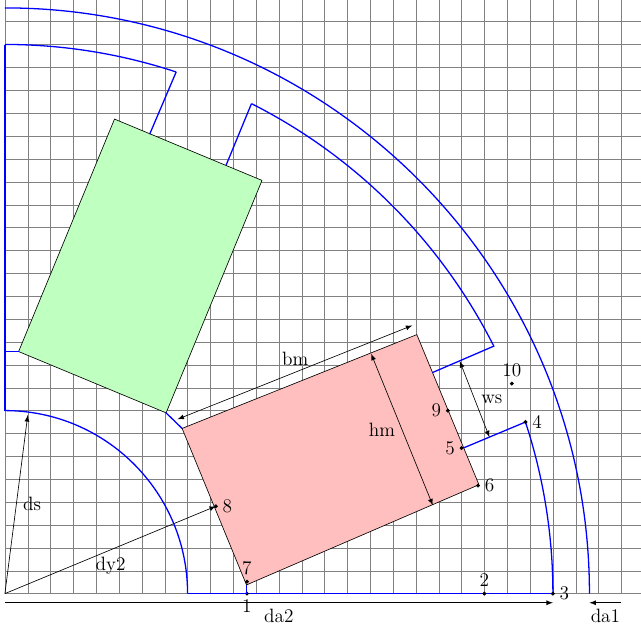Example of a user specified geometry (created with TikZ)

The steps are:

1. Create a diagram of the geometry and decide about the parameters to be used.

2. Create a fsl mako template file with the chosen parameters as placeholders using the Mako syntax with \${}.

3. Include this filename in the magnet model within the section using the basename of the mako file and set the parameter values.

The following values are defined globally and can be referenced in the template:

Name

Description

Unit

mcvkey_yoke

lamination material of yoke

mcvkey_shaft

material of shaft

m.remanenc

Remanence Induction

T

m.relperm

Rel. Permeability of PM

m.num_poles

Number of poles

m.npols_gen

Number of poles in model

agndst

Node distance in airgap

m

dy1

Diameter of stator yoke

m

da1

Diameter of stator bore

m

da2

Diameter of rotor

m

dy2

Diameter of rotor yoke

m

Example FSL Template file ‘’spoke.mako’’ using 4 parameters:

```-- Model parameters

ds = \${model['shaft_diam']}*1e3
hm = \${model['magn_height']}*1e3
bm = \${model['magn_width']}*1e3
ws = \${model['slot_width']}*1e3

-- calculate slot height and pole pitch
hs = (da2-dy2)/2 - bm
taup = math.pi/m.num_poles

x = {}
y = {}

-- characteristic points of model

ar = math.sqrt(dy2^2+hm^2)/2
x = ar*math.cos(taup - math.atan2(hm/2, dy2/2))
y = 0
x,y = pr2c(dy2/2+bm, 0)
x,y = pr2c(da2/2, 0)
x,y = pr2c(da2/2, taup - math.atan2(ws/2,(da2/2)))
x,y = pr2c(da2/2-hs, taup - math.atan2(ws/2,(da2/2 - hs)))
x,y = pr2c(da2/2-hs, taup - math.atan2(hm/2, da2/2-hs))
x,y = pr2c(ar, taup - math.atan2(hm/2, dy2/2))
x,y = pr2c(dy2/2, taup)
x,y = pr2c(da2/2 - hs, taup)
x,y = pr2c(da2/2, taup)
```

Magnet definition in Python file with included mako file (eg in this case ‘’spoke’’) and the parameter definition:

```..
magnet=dict(
mcvkey_yoke="dummy",
spoke=dict(
magn_height=0.008,
shaft_diam=0.01,
slot_width=0.004,
magn_width=0.024
)
..
```

The resulting model: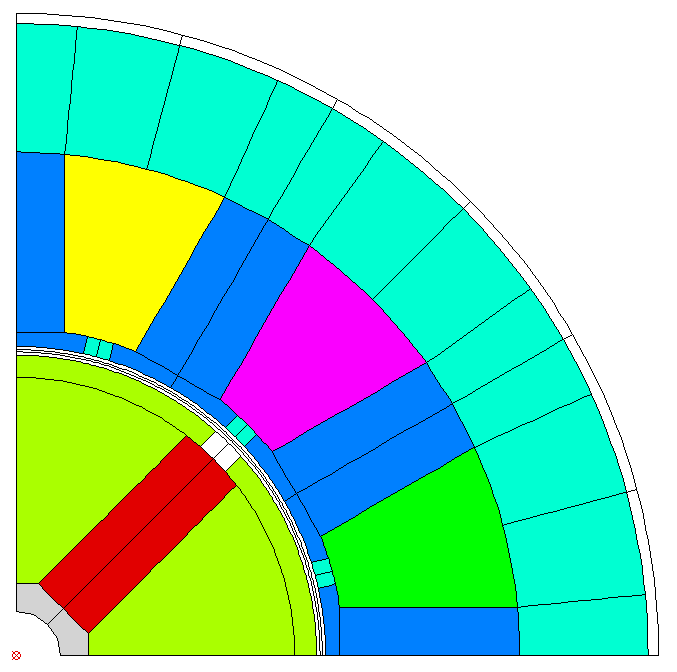Note

Starting with Release 0.4.4 the syntax has changed but backward compatibility is fully supported.

the complete example can be found in stator1-spoke.py and spokefml.mako in the example directory on github.

## FE-Simulation¶

Cogging (cogg_calc)

Parameter

Description

Default

Unit

speed

Speed

1/s

skew_angle

Skewing angle

0

deg

num_skew_steps

Number of skew steps

0

magn_temp

Magnet Temperature

°C

num_move_steps

Number of move steps

num_par_wdgs

Number of parallel windings

1

eval_force

Evaluate force

0

period_frac

Rotate Fraction of Period

1

vtu_movie

Create VTU files

False

Example:

```operatingConditions = dict(
calculationMode="cogg_fast",
magn_temp=60.0,
num_move_steps=49,
speed=50.0)
```

PM/Rel Machine Simulation (pm_sym_fast)

Parameter

Description

Default

Unit

speed

Speed

1/s

skew_angle

Skewing angle

0

deg

num_skew_steps

Number of skew steps

0

magn_temp

Magnet Temperature

°C

wind_temp

Winding Temperature

20

°C

num_move_steps

Number of move steps

49

num_par_wdgs

Number of parallel windings

1

eval_force

Evaluate force

0

explicit_mode

0

current

Phase current

A (RMS)

angl_i_up

Angle I vs. Up

0

deg

optim_i_up

Optimize Current

0

phi_start

Start angle of rotation

0

deg

range_phi

Rotation angle

360/p

deg

explicit_mode

Deactivate rotation correction

0

plots

Create plots

[]

airgap_induc

calculate airgap induction

False

period_frac

Rotate Fraction of Period

1

vtu_movie

Create VTU files

False

Note

plots is a list of field_lines or color_gradation plots to be created after the calculation. Possible values ‘field-lines’, ‘Babs’, ‘Br’, ‘Bx’, ‘By’, ‘Br’, ‘Bt’, ‘Habs’, ‘Hx’, ‘Hy’, ‘Hr’, ‘Ht’ ‘demag’, ‘ecurr’, ‘ecloss’, ‘relperm’, ‘Wm’, ‘Bdev’, ‘Vpot’. (See http://script.profemag.ch/ColorGrad.html) added in version 0.0.16. The value types can be simple strings or list with name and min/max range.

Example:

```operatingConditions = dict(
calculationMode="pm_sym_fast",
wind_temp=60.0,
magn_temp=60.0,
current=50.0,
speed=50.0,
plots=['field_lines', ['Babs', 0.0, 2.5]])
```

Note

If airgap_induc is True the induction in the airgap is calculated after the simulation returns. The values can be read with the method read_airgap_induc() of call Femag.

Parameter

Description

Unit

Baml

Amplitude of base harmonic

T

phi0

Phase angle of base harmonic

pos

Position

° or mm

B

sampled values

T

B_fft

Values of base harmonic

T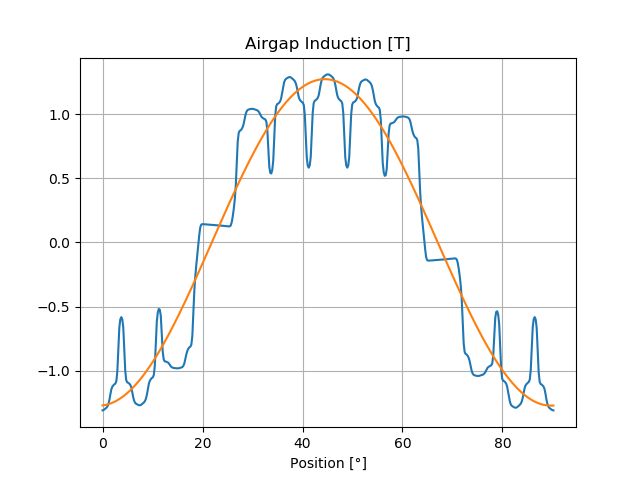Short circuit calculation (shortcircuit)

The short circuit calculation is executed subsequentially to a pm_sym_fast simulation if shortCircuit is set True. (version added 0.9.30). The results are included in scData dict of bch

Parameter

Description

Default

Unit

shortCircuit

run short circuit calc if True

l_end_winding

winding inductance

0

H

l_external

External inductance

0

H

sc_type

type of short circuit (3-phase)

3

simultime

Simulation time

0.1

s

initial

2

allow_demagn

Allow Demagnetisation:1:yes; 0:no

0

sim_demagn

Simulate Demagnetisation:1:yes; 0:no

0

Example:

```pmRelSim = dict(
angl_i_up=-39.3,
calculationMode="pm_sym_fast",
wind_temp=60.0,
magn_temp=60.0,
current=76.43,
period_frac=6,
speed=50.0,
shortCircuit=True,
l_end_winding=0,
l_external=0,
sc_type=3,
initial=2,
allow_demagn=0,
sim_demagn=1)

r = femag(machine,
pmRelSim)

print('Torque [Nm] = {}'.format(r.machine['torque']))
print('''
Short Circuit    Current         Torque
Peak       iks {2:8.1f} A  tks {3:8.1f} Nm
Stationary ikd {0:8.1f} A  tkd {1:8.1f} Nm

peak winding currents {4}
'''.format(r.scData['ikd'],
r.scData['tkd'],
r.scData['iks'],
r.scData['tks'],
r.scData['peakWindingCurrents']))

fig, ax = plt.subplots()
femagtools.plot.transientsc(r)
plt.show()
```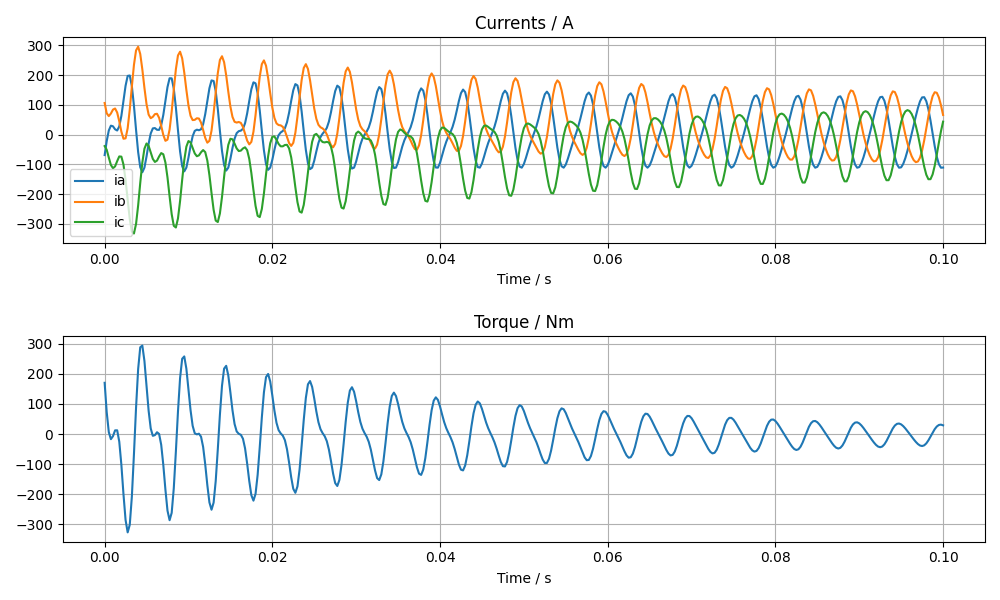Ld-Lq Identification (ld_lq_fast)

Parameter

Description

Default

Unit

speed

Speed

1/s

skew_angle

Skewing angle

0

deg

num_skew_steps

Number of skew steps

0

magn_temp

Magnet Temperature

°C

num_move_steps

Number of move steps

num_par_wdgs

Number of parallel windings

1

eval_force

Evaluate force

0

i1_max

Max. phase current

A (RMS)

beta_min

Min. Beta angle

deg

beta_max

Max. beta angle

deg

num_cur_steps

Number of current steps

num_beta_steps

Number of beta steps

period_frac

Rotate Fraction of Period

1

Example:

```feapars = dict(
num_move_steps=25,
calculationMode="ld_lq_fast",
magn_temp=60.0,
i1_max=150.0,
beta_max=0.0,
beta_min=-60.0,
num_cur_steps=3,
num_beta_steps"=4,
speed=50.0)
```

Psid-Psiq Identification (psd_psq_fast)

Parameter

Description

Default

Unit

speed

Speed

1/s

skew_angle

Skewing angle

0

deg

num_skew_steps

Number of skew steps

0

magn_temp

Magnet Temperature

°C

num_move_steps

Number of move steps

num_par_wdgs

Number of parallel windings

1

eval_force

Evaluate force

0

maxid

Max. Amplitude Id current

A

minid

Min. Amplitude Id current

A

maxiq

Max. Amplitude Iq current

A

miniq

Min. Amplitude Iq current

A

delta_id

Delta of Id current steps

A

delta_iq

Delta of Iq current steps

A

period_frac

Rotate Fraction of Period

1

Example:

```feapars = dict(
num_move_steps=25,
calculationMode="psd_psq_fast",
magn_temp=60.0,
maxid=0.0,
minid=-150.0,
maxiq=150.0
miniq=0.0,
delta_id=50.0,
delta_iq=50.0,
speed=50.0)
```

PM/Rel Torque Calc (torq_calc)

Parameter

Description

Default

Unit

speed

Speed

1/s

skew_angle

Skewing angle

0

deg

num_skew_steps

Number of skew steps

0

magn_temp

Magnet Temperature

°C

wind_temp

Winding Temperature

20

°C

num_move_steps

Number of move steps

49

num_par_wdgs

Number of parallel windings

1

current

Phase current

A (RMS)

angl_i_up

Angle I vs. Up

0

deg

Example:

```operatingConditions = dict(
calculationMode="torq_calc",
wind_temp=60.0,
magn_temp=60.0,
current=50.0,
angl_i_up=0.0,
speed=50.0)
```

### FE-Simulation with existing model¶

FE calculations can be executed for existing models also. Since Femag Rel 8.3 there is no need to fully specify the machine model:

```machine = "PM 270 L8"

workdir = os.path.join(
os.path.expanduser('~'), 'femag')

femag = femagtools.Femag(workdir)

operatingConditions = dict(
angl_i_up=-38.7,
calculationMode="pm_sym_fast",
magn_temp=60.0,
num_move_steps=25,
speed=50.0,
wind_temp=60.0,
current=108.0)

r = femag(machine,
operatingConditions)
```

For older FEMAG versions the minimal data is:

```machine = dict(
name="PM 130 L4",
lfe=0.1,
poles=4,
outer_diam=0.13,
bore_diam=0.07,
airgap=0.001,

stator=dict(
num_slots=12,
num_slots_gen=3,
mcvkey_yoke="dummy"
)
)
```

## Model Creation with DXF¶

The goal of the dxfsl modules is to create a complete FE model for rotating PM and Reluctance Machines on the basis of a DXF file with as little restrictions as possible. This has the consequence that symmetries, subregions, magnets, windings, boundary conditions need to be identified.

The procedure is as follows:

1. Read the DXF file and create a graph object with nodes and edges.

2. Identify the areas stator, rotor and airgap and their symmetry axis.

3. Add auxiliary lines if required by the meshing process.

4. Convert the graph object into FSL code including the identified subregions.

For monitoring and trouble-shooting purposes it is possible to create plots that display the intermediate results.

Example with a single dxf file motor.dxf:

```machine = dict(
name="Motor",
lfe=0.001,

dxffile=dict(
name='motor.dxf'
),
stator=dict(
mcvkey_yoke='dummy',
mcvkey_shaft="dummy"
),
magnet=dict(
mcvkey_yoke="dummy",
mcvkey_shaft="dummy"
),
...
```

Note

The parameters poles, outer_diam, bore_diam and airgap as well as num_slots and num_slots_gen will be set automatically

Example with two separate dxf files for stator and rotor:

```machine = dict(
name="Motor",
lfe=0.001,

stator=dict(
mcvkey_yoke='dummy',
dxffile=dict(
name='mystator.dxf',
position='out',
split=True
)
),
magnet=dict(
mcvkey_yoke="dummy",
mcvkey_shaft="dummy",
dxffile=dict(
name='myrotor.dxf',
position='in',
split=True
)
),
...
```